# Clamping Force Calculations

Clamping-force calculations can be quite complicated. Sometimes an approximate method is good enough. See table below for how much clamping force is available from manual clamp straps of various sizes (with a 2-to-1 clamping-force ratio) to compare with power-clamp forces.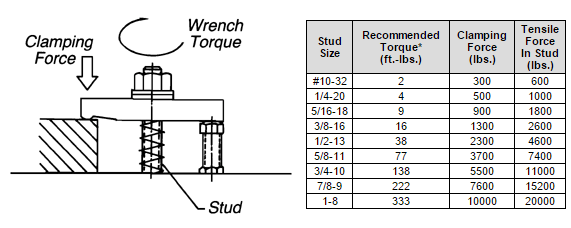*Clean, dry clamping stud torqued to approximately 33% of its 100,000 psi yield strength (2:1 lever ratio).

You can also calculate required clamping forces based on calculated cutting force. A simplified example appears below, with cutting force entirely horizontal, and no workpiece stops (frictional force resists the entire cutting force).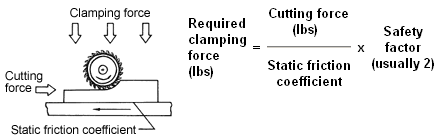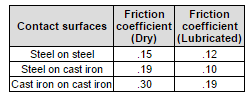With workpiece stops and multi-direction forces, calculations become much more complicated. To simplify somewhat, determine the worst-case force situation intuitively, then treat the calculation as a two-dimensional static-mechanics problem (using a free-body diagram). In the example below, cutting force is already known to be 1800 lbs from previous calculations. The workpiece weighs 1500 lbs.Unknown forces are:
 FR = Total force from all clamps on right side FL = Total force from all clamps on left side R1 = Horizontal reaction force from fixed stop R2 = Vertical reaction force from fixed stop R3 = Vertical reaction force on right side N = Normal force = FL + FR + 1500 µ = = Coefficient of friction = .19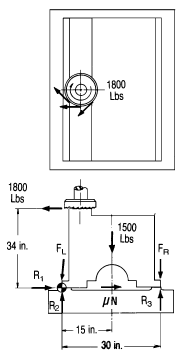The equations below solve for unknown forces assuming that for a static condition:

1. The sum of forces in the x direction must equal zero
2. The sum of forces in the y direction must equal zero
3. The sum of moments about any point must equal zero

At first glance, the example above looks "statically indeterminate," i.e. there are 5 variables and only 3 equations. But for the minimum required clamping force, R3 would be zero (workpiece barely touching) and FL would be zero (there is no tendency to lift on the left side). Now with only 3 variables, we can solve: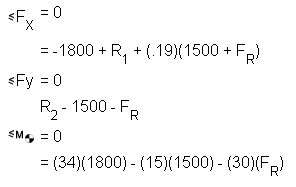Solving for the variables,

FR = 1290 lbs
R1 = 1270 lbs
R2 = 2790 lbs

In other words, the combined force from all clamps on the right side must be greater than 1290 lbs. We recommend a 2-to-1 safety factor (2580 lbs). Even though FL (the combined force from all clamps on the left side) equals zero, a small clamping force may be desirable to prevent vibration.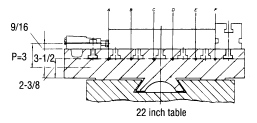Too much clamping force can be as bad as too little. Excess force can cause fixture and machine-table distortion or even damage. Even a small hydraulic clamp can generate tremendous stresses (S).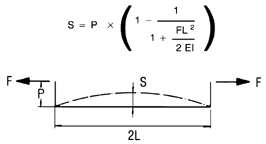In the example above, three 4560-lb Edge Clamps cause some machine-table bending. Using static beam-binding calculations, maximum distortion, at point D, is about .0006 inches (probably acceptable). However, if the clamping point were higher off the machine table (P dimension), distortion would be much greater. Higher clamps would require adding an intermediate fixture plate to increase table rigidity.